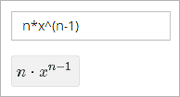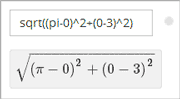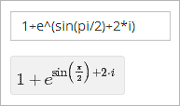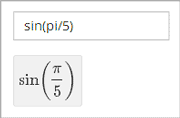# 12.1. Completing Mathematical and Scientific Assignments#

This topic describes how to enter mathematical and scientific expressions for problems in your edX course.

## 12.1.1. Overview of Mathematical and Scientific Expressions#

You might want to enter a mathematical or scientific expression into an assignment in the body of your course, into the edX calculator tool, or into a course discussion.

For assignments in the body of the course and for the calculator tool, you enter plain text, and the edX system then converts your text into numbers and symbols that appear below the response field. For more information, see Entering Math Expressions in Assignments or the Calculator.For course discussions, you use MathJax to format the text that you type, and the system then converts your text into a mathematical expression. For more information, see Entering Math Expressions in Course Discussions.

## 12.1.2. Completing Mathematical Problems with Screen Readers#

EdX has carefully designed assessments that are accessible to screen readers. However, because of a limitation with HTML and screen reader technology, screen readers may not be able to read math problems in edX courses by default.

To access these problems with a screen reader, use one of the following methods.

• If your browser and screen reader both support MathML, specify MathML as your preferred math renderer in MathJax.

Note

• In Interactive mode, learners use the Tab key to move from one interactive element to the next.

• In Reading mode, learners use the arrow keys to read all of the content in a document, and not just the interactive elements.

## 12.1.3. Entering Math Expressions in Assignments or the Calculator#

Both the calculator and the response fields in math problems accept a selection of characters that represent numbers, operators, constants, functions, and other mathematical concepts. You might recognize parts of this system if you have used math programs before.

Note

If your course offers the calculator tool, the calculator appears as a small icon on all pages in the body of the course. To open the calculator, select the calculator icon. To close the calculator, select the X that appears when the calculator is open.The calculator includes an information page that shows an abbreviated version of the information in this topic. To see the information page, select the circled i icon next to the input field.When you enter your plain text into the calculator or the response field, follow these guidelines.

• Arithmetical operations: Use standard characters for addition (+), subtraction (-), multiplication (*), and division (/).

• Multiplication: Be sure to indicate multiplication explicitly. That is, instead of mc^2 type m*c^2, and instead of 5a+4b+3c type 5*a+4*b+3*c.

• Operation order: Use parentheses (( )) to specify the order of operations and to make your expression as clear as possible. Use curved parentheses (( )) only. Do not use brackets ([ ]) or braces ({ }).

• Exponents or superscripts: Insert a caret (^) before an exponent or superscript. If the exponent or superscript includes multiple characters or is an expression, surround the expression with parentheses. For example, you can enter x^n or x^(n-1).

• Subscripts: Insert an underscore (_) before a subscript to indicate a subscript. If the subscript has multiple characters, type the characters without a space. For example, you can enter v_IN-v_OUT. Note, however, that subscripts cannot currently include operators or parentheses.

• Avoid whitespace.

• Do not use equal signs (=).

• Because the system is case-sensitive, make sure you use uppercase and lowercase letters carefully.

Note

The edX system accepts both constants and metric affixes. Be careful to distinguish between constants and metric affixes. Constants stand alone, while metric affixes must be combined with numbers.

For example, c can be a constant representing the speed of light or a metric affix meaning “centi”. When you use c as a metric affix, do not include a space between c and the number. When you use c as a constant, indicate multiplication explicitly. The following examples show the difference:

• 2c = 0.02 (2 multiplied by 0.01)

• 2*c = 599584916.0 (the speed of light multiplied by 2)

• 2M = 2,000,000 (2 multiplied by 1,000,000)

• 2*M = 2 multiplied by the variable M

### 12.1.3.1. Numbers#

You can use the following types of numbers.

• Integers: 2520

• Fractions: 2/3

• Normal floats: 3.14

• Floats with no integer part: .98

The largest number you can use is 1.7977e+308, which is the largest float possible in the Python programming language.

### 12.1.3.2. Scientific Notation and Metric Affixes#

You can enter metric affixes or scientific notation to indicate very large or very small numbers. For scientific notation, you can type either a caret (^) or the letter e followed by a number to indicate an exponent. You can use both positive and negative exponents.

For example, to indicate 0.012, you can enter either of the following expressions:

• 1.2*10^-2

• 1.2e-2

To indicate -440,000, you can enter either of the following expressions:

• -4.4*10^5

• -4.4e5

The following table shows how to enter numbers with metric affixes, with scientific notation, and with e notation.

To enter this number

Use this metric affix

Use this scientific notation

Use this e notation

Other notation

0.1

1d (deci)

10^-1

1e-1

0.01

1c (centi)

10^-2

1e-2

1% (percent)

0.001

1m (milli)

10^-3

1e-3

0.000001

1u (micro)

10^-6

1e-6

0.000000001

1n (nano)

10^-9

1e-9

0.000000000001

1p (pico)

10^-12

1e-12

1000

1k (kilo)

10^3

1e3

1,000,000

1M (mega)

10^6

1e6

1,000,000,000

1G (giga)

10^9

1e9

1,000,000,000,000

1T (tera)

10^12

1e12

Note

When you use metric affixes or e notation, make sure you do not include spaces between the number and the metric affix or the e.

### 12.1.3.3. Constants#

You can use several different constants in your mathematical expressions.

Note

When you enter constants multiplied by a number, make sure to indicate the multiplication explicitly. For example, enter 2*c instead of 2c and -4*i instead of -4i.

Constant

Value

c

The speed of light in m/s (2.998^8)

e

Euler’s number (2.718…)

g

Gravity (9.80 m/s^2)

i

The square root of -1

j

The square root of -1

k

The Boltzmann constant (~1.38^-23 in Joules/Kelvin)

pi

The ratio of a circle’s circumference to its diameter (3.14159…)

q

The fundamental charge (~1.602^-19 Coloumbs)

T

The positive difference between 0K and 0°C (273.15)

### 12.1.3.4. Greek Letters#

To use any of the following Greek letters, type the name of the letter in the calculator or the response field.

Name

Letter

alpha

α

beta

β

gamma

γ

delta

δ

epsilon

ϵ

varepsilon

ε

zeta

ζ

eta

η

theta

θ

vartheta

ϑ

iota

ι

kappa

ϰ

lambda

λ

mu

μ

nu

ν

xi

ξ

pi

π

rho

ρ

sigma

σ

tau

τ

upsilon

υ

phi

ϕ

varphi

φ

chi

χ

psi

ψ

omega

ω

### 12.1.3.5. Functions#

To use a function, type the letters that represent the function, and then surround the expression in that function with parentheses. For example, to represent the square root of 4*a+b, type sqrt(4*a+b).

You can use the following functions.

• Common functions

• sqrt

• log10

• log2

• ln

• exp

• abs

• Trigonometric functions and their inverses, as well as hyperbolic trigonometric functions and their inverses.

Function

Inverse

Hyperbolic Function

Inverse

sin

arcsin

sinh

arcsinh

cos

arccos

cosh

arccosh

tan

arctan

tanh

arctanh

sec

arcsec

sech

arcsech

csc

arccsc

csch

arccsch

cot

arccot

coth

arccoth

• Factorials: Enter factorials as fact(3) or factorial(3). You must use integers. For example, you cannot enter fact(1.5).

• A “parallel resistors” operator (||). For example, 1 || 2 represents the resistance of a pair of parallel resistors (of resistance 1 and 2 ohms), evaluating to 2/3 (ohms).

## 12.1.4. Entering Math Expressions in Course Discussions#

Entering math expressions in course discussions is different from entering math expressions in a math problem or in the calculator. In course discussions, you use MathJax to format the text that you type.

For detailed information about how to enter math expressions in course discussions, see Math Formatting in Course Discussions.